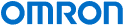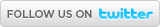Industrial Automation | Europe

# OMRON News

## 08/08/2023 - Innovation boost for e-mobility: OMRON new member at renowned research campus ARENA203607/08/2023 - Introducing the OMRON V450-H DPM handheld scanner12/06/2023 - OMRON launches the M1 integrated global AC drives solution02/06/2023 - OMRON unveils the new ultra-compact MicroHAWK F440-F 5MP C-Mount Smart Camera23/05/2023 - OMRON launches new G2RV-ST industrial 6mm relay# myKnowledge

Main > Product Type > Automation Systems > PLCsPrintE-mail
 English Czech Danish Dutch French Finnish German Hungarian Italian Japanese Norwegian Polish Portugese Romanian Russian Spanish Swedish Turkish

# Handling Programs That Have BCD Subtraction Operations That Result In A Negative Number

##### Introduction
When the result of a BCD arithmetic operation is a negative number, the operation result will be output as the 10’s complement (see Additional Information, below), and the Carry Flag will be turned ON. As shown in Figure 1, immediately after executing the BCD SUBTRACTION WITHOUT CARRY (-B) instruction, the status of the Carry Flag (CY) (ON or OFF) is checked.

a. When the Carry Flag (CY) Is OFF (Operation Result Is Positive)

The execution result (D202) of the BCD SUBTRACTION WITHOUT CARRY (-B) instruction is handled as an absolute value without any further manipulation.
In this case, the sign bit (CIO 2.00) turned OFF to indicate a positive number.

b. When the Carry Flag (CY) Is OFF (Operation Result Is Negative).

Subtract the execution result (D202: 10’s compliment) of the BCD SUBTRACTION WITHOUT CARRY (-B) instruction from 0 to convert it to the actual value, and store it in D202.
In this case, the sign bit (CIO 2.00) is turned ON to indicate a negative number.

What Is a Ten's Complement?

a. Subtract a 4-digit BCD value from 9,999 or an 8-digit BCD value from 99,999,999. The ten's complement is the result with 1 added. In Figure 1, the result of 6,666 - 9,999 is -3,333, but the result that is output is the ten’s complement of 3,333 (9,999 is -3,333 + 1, or 6,667). The formula for a 4-digit BCD number is written as follows:

Complement B = 9,999 - True value A + 1 (i)

b.Formula (i) can be reversed to calculate the true value A

True value A = 10,000 - Complement B (ii)

c.The ten's complement (6,667) can be converted to the true value A (3,333) using the following formula.

10,000 - 6,667 = 3,333. The true value of complement 6,667 is 3,333. (iii)

*[-B #0 D202 D202] in Figure 1 subtracts the complement from 0000, and stores the result in D202.The result of the first -B instruction is, 9,999 (D200) - 6,666 (D201) = 3333 (D202) and the Carry Flag (CY) is turned OFF. For this reason, the second -B instruction will not be executed, and the operation result is 3,333 is handled as an absolute value without any further manipulation. In this case, the sign bit (CIO 2.00) is turned OFF to indicate a positive number.

BCD SUBTRACT WITHOUT CARRY (-B) Instruction
Word operands and/or constants are subtracted as 4-digit BCD values.

Carry Flag (CY)
This flag is turned ON if there is a carry in the result of the subtraction.Operation
The result of the first -B instruction execution is, 6,666 (D200) - 9,999 (D201) = 6667 (the ten's compliment of 3,333 (D202)) and the Carry Flag (CY) is turned ON. The second -B instruction will be executed, and the complement of 6,667 is converted to the true value (3,333) and stored in D202. In this case, the sign bit (CIO 2.00) is turned ON to indicate a negative number.